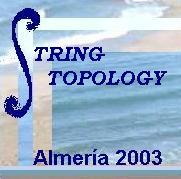## Summaries of courses

See these summaries as a PDF file.

### String Topology and Beyond, by Alexander A. Voronov

String Topology is a new field of Algebraic and Geometric Topology suggested or motivated by Mathematical Physics, in particular, Quantum Field Theory and String Theory. This field was opened to the mathematics community by a pioneering work of Chas and Sullivan, String Topology, to appear in the Annals of Mathematics [CS]. Further development came through a number of papers, including those of Chas [C], Cohen and Jones [CJ], Cattaneo, Froehlich, and Pedrini [CFP], Kisisel [K], Kaufmann, Livernet, Penner [KLP], and current work of Sullivan and the lecturer; see [V]. The field is developing rapidly and is at a lucky stage, when it appears that the more the field develops, the more exciting problems it opens.

String Topology uses ideas of sigma-model (a two-dimensional quantum field theory) to introduce new algebraic structure on the homology of a free loop space. This algebraic structure is similar to the celebrated quantum cohomology and Gromov-Witten invariants, but done in a purely topological setting. In a way, in String Topology one constructs fusion rules and the whole structure of a 2d quantum field theory on a loop space. Loop spaces have long been very important objects of Algebraic Topology and studied very extensively. One of the reasons for the current excitement about String Topology is that it tells new things about these old objects. Further study of String Topology had brought and will undoubtedly bring powerful new results in Algebraic Topology and Mathematical Physics.

(Visit Voronov's homepage: http://www.math.umn.edu/~voronov/)

### String Topology, Graphs and Morse Theory, by Ralph Cohen

In these lectures I will survey several aspects of string topology. Topics will be chosen from the following:

- Multiplicative constructions on Thom spectra of bundles over the loop space. The relation to topological Hochschild (co)homology.
- Is string topology a homotopy invariant?
- Applications of spaces of graphs to parameterize string topology operations. What we know and don't know about the field theoretic properties of string topology.
- Operations on open strings.
- A Morse theoretic viewpoint of string topology. Relation to Gromov-Witten theory.

(Visit Cohen's homepage: http://math.stanford.edu/~ralph/)

### Hochschild and cyclic homology and its relation with free loop spaces, by Kathryn Hess

Hochschild and cyclic homologies are in some strong sense additive versions of algebraic K-theory [Lo]. In this way computing these homology theories is fundamental. But it is also strongly related to non-commutative geometry and the deep algebraic structure underlying the Hochschild cochain complex (the so called Deligne's conjecture) is one of the main ingredient in Kontsevich's proof of deformation by quantification of Poisson manifolds.

Also motivated by computations in algebraic K-theory, Bokstedt, Hsiang, Madsen have introduced Topological Hochschild and Topological cyclic homology [BHM]. These two theories have been a wonderful technical tool to compute some K-groups [HM].

Recently borrowing ideas from rational homotopy theory Hess and Rognes have given algebraic models for computing Topological Hocschild and cyclic homology. These models are based on previous work on algebraic models for homotopy pullbacks by Dupont and Hess [DH] in particular free loop spaces [DH2]. This is also related to the closed geodesics problem [SV].

(Visit Hess's homepage: http://dmawww.epfl.ch/~hess/)

### REFERENCES

[BHM] M. BOKSTEDT; W. C. HSIANG; I. MADSEN, The cyclotomic trace and algebraic \$K\$-theory of spaces, Invent. Math. 111 (1993), no. 3, 465--539.

[CFP] A. S. CATTANEO, J. FROEHLICH, B. PEDRINI, Topological Field Theory Interpretation of String Topology, math.GT/0202176.

[C] M. CHAS, Combinatorial Lie bialgebras of curves on surfaces, math.GT/0105178.

[CS] M. CHAS, D. SULLIVAN, String topology, math.GT/9911159, to appear in Annals of Mathematics.

[CJ] R. COHEN, J. D.S. JONES, A homotopy theoretic realization of string topology, Math. Ann. 324 (2003), no. 4, 773--798, math.GT/0107187

[DH] N. DUPONT, K. HESS, Noncommutative algebraic models for fiber squares, Math. Ann. 314 (1999), no. 3, 449--467.

[DH2] N. DUPONT, K. HESS, How to model the free loop space algebraically, Math. Ann. 314 (1999), no. 3, 469--490.

[G] E. GETZLER, Operads and Moduli spaces of genus zero Riemann surfaces,
in: The Moduli Spaces of Curves ed. by R. Dijkgraaf, C. Faber, G. Van der Geer, Progress in Math., vol. 129 Birkhauser 1995, 199-230.

[HM] L. HESSELHOLT; I. MADSEN, Cyclic polytopes and the \$K\$-theory of truncated polynomial algebras, Invent. Math. 130 (1997), no. 1, 73--97.

[HGE] H. HOFER, A. GIVENTAL, Y. ELIASHBERG, Introduction to Symplectic Field Theory, math.SG/0010059

[KLP] R. M. KAUFMANN, M. LIVERNET, R. C. PENNER, Arc Operads and Arc Algebras, math.GT/0209132.

[K] A. U. O. KISISEL, The Hamiltonian structure of discrete KP equations, math-ph/0102025.

[Lo] J.L. LODAY, Cyclic homology. Appendix E by María O. Ronco. Second edition. Chapter 13 by the author in collaboration with Teimuraz Pirashvili.} Grundlehren der Mathematischen Wissenschaften [Fundamental Principles of Mathematical Sciences], 301. Springer-Verlag, Berlin, 1998. xx+513 pp.

[SV] M. VIGUÉ-POIRRIER, D. SULLIVAN, The homology theory of the closed geodesic problem, J. Differential Geometry 11 (1976), no. 4, 633--644.

[V] A. A. VORONOV, Notes on universal algebra, math.QA/0111009, to appear in proceedings of Stony Brook conference on "Graphs and Patterns in Mathematics and Physics", June 2001.